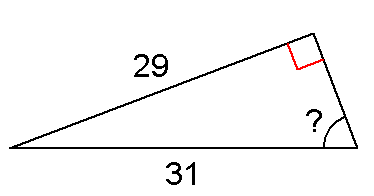X200 Identify Trigonometric Ratio Mav

12 Questions | Total Attempts: 62SettingsFind the appropriate ratio that will solve the triangl

• 1.
Which ratio should you use to solve the above triangle?
• A.

Sine

• B.

Cosine

• C.

Tangent

• D.

Sine, Cosine & Tangent could all work

• E.

None of these will solve this triangle

• 2.
Which ratio should you use to solve the above triangle?
• A.

Sine

• B.

Cosine

• C.

Tangent

• D.

Sine, Cosine & Tangent could all work

• E.

None of these will solve this triangle

• 3.
Which ratio should you use to solve the above triangle?
• A.

Sine

• B.

Cosine

• C.

Tangent

• D.

Sine, Cosine & Tangent could all work

• E.

None of these will solve this triangle

• 4.
Which ratio should you use to solve the above triangle?
• A.

Sine

• B.

Cosine

• C.

Tangent

• D.

Sine, Cosine & Tangent could all work

• E.

None of these will solve this triangle

• 5.
Which ratio should you use to solve the above triangle?
• A.

Sine

• B.

Cosine

• C.

Tangent

• D.

Sine, Cosine & Tangent could all work

• E.

None of these will solve this triangle

• 6.
Which ratio should you use to solve the above triangle?
• A.

Sine

• B.

Cosine

• C.

Tangent

• D.

Sine, Cosine & Tangent could all work

• E.

None of these will solve this triangle

• 7.
Which ratio should you use to solve the above triangle?
• A.

Sine

• B.

Cosine

• C.

Tangent

• D.

Sine, Cosine & Tangent could all work

• E.

None of these will solve this triangle

• 8.
Which ratio should you use to solve the above triangle?
• A.

Sine

• B.

Cosine

• C.

Tangent

• D.

Sine, Cosine & Tangent could all work

• E.

None of these will solve this triangle

• 9.
Which ratio should you use to solve the above triangle?
• A.

Sine

• B.

Cosine

• C.

Tangent

• D.

Sine, Cosine & Tangent could all work

• E.

None of these will solve this triangle

• 10.
Which ratio should you use to solve the above triangle?
• A.

Sine

• B.

Cosine

• C.

Tangent

• D.

Sine, Cosine & Tangent could all work

• E.

None of these will solve this triangle

• 11.
Which ratio should you use to solve the above triangle?
• A.

Sine

• B.

Cosine

• C.

Tangent

• D.

Sine, Cosine & Tangent could all work

• E.

None of these will solve this triangle

• 12.
Which ratio should you use to solve the above triangle?
• A.

Sine

• B.

Cosine

• C.

Tangent

• D.

Sine, Cosine & Tangent could all work

• E.

None of these will solve this triangle

• 13.
Which ratio should you use to solve the above triangle?
• A.

Sine

• B.

Cosine

• C.

Tangent

• D.

Sine, Cosine & Tangent could all work

• E.

None of these will solve this triangle

• 14.
Which ratio should you use to solve the above triangle?
• A.

Sine

• B.

Cosine

• C.

Tangent

• D.

Sine, Cosine & Tangent could all work

• E.

None of these will solve this triangle

• 15.
Which ratio should you use to solve the above triangle?
• A.

Sine

• B.

Cosine

• C.

Tangent

• D.

Sine, Cosine & Tangent could all work

• E.

None of these will solve this triangle

• 16.
Which ratio should you use to solve the above triangle?
• A.

Sine

• B.

Cosine

• C.

Tangent

• D.

Sine, Cosine & Tangent could all work

• E.

None of these will solve this triangle

• 17.
Which ratio should you use to solve the above triangle?
• A.

Sine

• B.

Cosine

• C.

Tangent

• D.

Sine, Cosine & Tangent could all work

• E.

None of these will solve this triangle

• 18.
Which ratio should you use to solve the above triangle?
• A.

Sine

• B.

Cosine

• C.

Tangent

• D.

Sine, Cosine & Tangent could all work

• E.

None of these will solve this triangle

• 19.
Which ratio should you use to solve the above triangle?
• A.

Sine

• B.

Cosine

• C.

Tangent

• D.

Sine, Cosine & Tangent could all work

• E.

None of these will solve this triangle

• 20.
Which ratio should you use to solve the above triangle?
• A.

Sine

• B.

Cosine

• C.

Tangent

• D.

Sine, Cosine & Tangent could all work

• E.

None of these will solve this triangle

• 21.
Which ratio should you use to solve the above triangle?
• A.

Sine

• B.

Cosine

• C.

Tangent

• D.

Sine, Cosine & Tangent could all work

• E.

None of these will solve this triangle

• 22.
Which ratio should you use to solve the above triangle?
• A.

Sine

• B.

Cosine

• C.

Tangent

• D.

Sine, Cosine & Tangent could all work

• E.

None of these will solve this triangle

• 23.
Which ratio should you use to solve the above triangle?
• A.

Sine

• B.

Cosine

• C.

Tangent

• D.

Sine, Cosine & Tangent could all work

• E.

None of these will solve this triangle

• 24.
Which ratio should you use to solve the above triangle?
• A.

Sine

• B.

Cosine

• C.

Tangent

• D.

Sine, Cosine & Tangent could all work

• E.

None of these will solve this triangle

• 25.
Which ratio should you use to solve the above triangle?
• A.

Sine

• B.

Cosine

• C.

Tangent

• D.

Sine, Cosine & Tangent could all work

• E.

None of these will solve this triangleBack to top